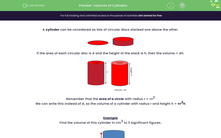# Calculate the Volumes of Cylinders

In this worksheet, students will calculate the volume of cylinders given their diameter and height.Key stage:  KS 3

Curriculum topic:   Geometry and Measures

Curriculum subtopic:   Use 3D Shape Knowledge to Solve Problems

Popular topics:   Geometry worksheets

Difficulty level:#### Worksheet Overview

A cylinder can be considered as lots of circular discs stacked one above the other.If the area of each circular disc is A and the height of the stack is h, then the volume = Ah.Remember that the area of a circle is πr2 where r is the radius of the circle.

So the volume of a cylinder with radius r and height h = π x r2 x  h or as πr2

Example

Find the volume of this cylinder in cm3 to 3 significant figures.Diameter = 6 cm

Radius = 6 ÷ 2 = 3 cm

Height = 12 cm

Volume = πr2h = π × 32 × 12 = π  x 9 x 12 = 339.29 ≈ 339 cm3 (3 s.f.)

Let's try some questions now.

### What is EdPlace?

We're your National Curriculum aligned online education content provider helping each child succeed in English, maths and science from year 1 to GCSE. With an EdPlace account you’ll be able to track and measure progress, helping each child achieve their best. We build confidence and attainment by personalising each child’s learning at a level that suits them.

Get started